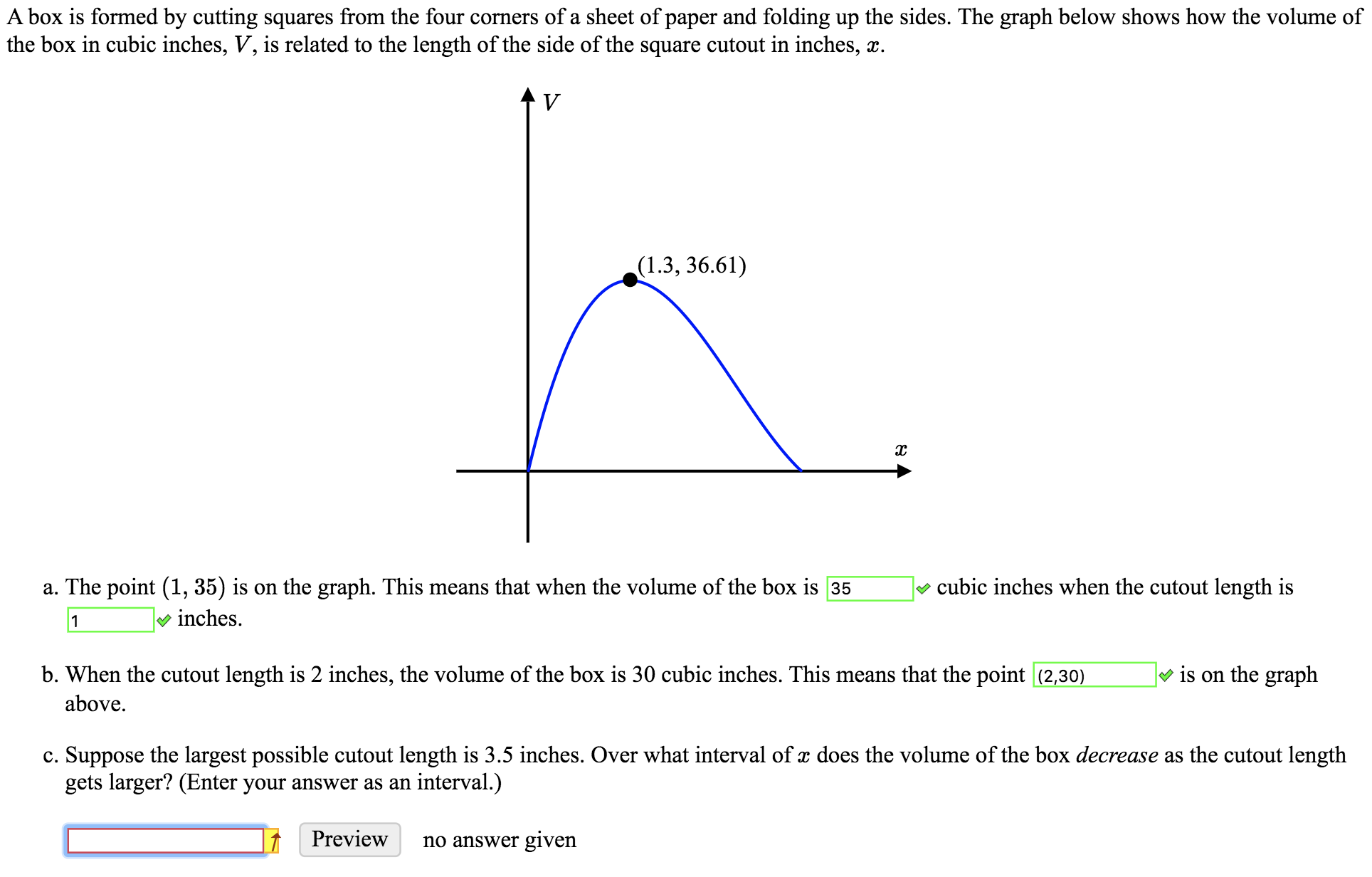# A box is formed by cutting squares from the four corners of a sheet of paper and folding up the sides. The graph below shows how the volume ofthe box in cubic inches, V, is related to the length of the side of the square cutout in inches, xV(1.3, 36.61)cubic inches when the cutout length isa. The point (1, 35) is on the graph. This means that when the volume of the box is 35inches.1is on the graphb. When the cutout length is 2 inches, the volume of the box is 30 cubic inches. This means that the point (2,30)abovec. Suppose the largest possible cutout length is 3.5 inches. Over what interval of x does the volume of the box decrease as the cutout lengthgets larger? (Enter your answer as an interval.)Previewno answer given

Question
367 viewshelp_outlineImage TranscriptioncloseA box is formed by cutting squares from the four corners of a sheet of paper and folding up the sides. The graph below shows how the volume of the box in cubic inches, V, is related to the length of the side of the square cutout in inches, x V (1.3, 36.61) cubic inches when the cutout length is a. The point (1, 35) is on the graph. This means that when the volume of the box is 35 inches. 1 is on the graph b. When the cutout length is 2 inches, the volume of the box is 30 cubic inches. This means that the point (2,30) above c. Suppose the largest possible cutout length is 3.5 inches. Over what interval of x does the volume of the box decrease as the cutout length gets larger? (Enter your answer as an interval.) Preview no answer given fullscreen
check_circle

Step 1

(c) It is given that the largest possible cut out length is 3.5 inches. That is the largest length is attained when V tends to zero.

Step 2

Clearly, from the graph it can be inferred that,...

### Want to see the full answer?

See Solution

#### Want to see this answer and more?

Solutions are written by subject experts who are available 24/7. Questions are typically answered within 1 hour.*

See Solution
*Response times may vary by subject and question.
Tagged in

### Coordinate Geometry